# A certain reaction has an energy change of AE = +29 kJ and an activation energy...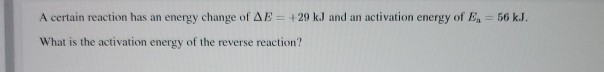A certain reaction has an energy change of AE = +29 kJ and an activation energy of B = 56 kJ. What is the activation energy of the reverse reaction?#### Earn Coin

Coins can be redeemed for fabulous gifts.

Similar Homework Help Questions
• ### gnment Score: 0% 01 49 0 Hint stion 3 of 20 > A certain reaction has...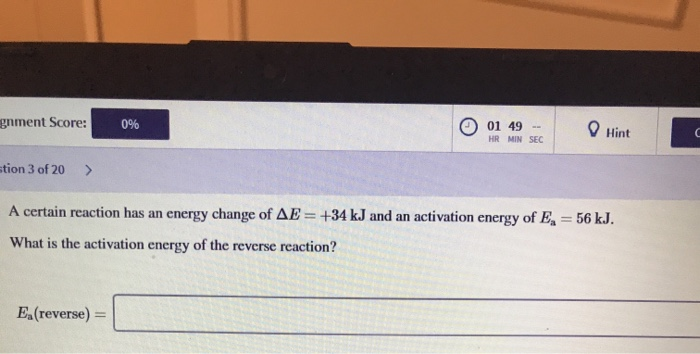gnment Score: 0% 01 49 0 Hint stion 3 of 20 > A certain reaction has an energy change of AE = +34 kJ and an activation energy of En = 56 kJ. What is the activation energy of the reverse reaction? E.(reverse) =

• ### A certain reaction has an enthalpy of AH = -49 kJ and an activation energy of...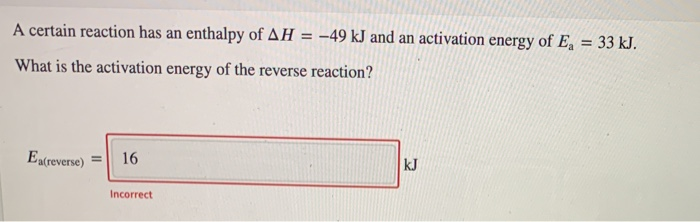A certain reaction has an enthalpy of AH = -49 kJ and an activation energy of E, = 33 kJ. What is the activation energy of the reverse reaction? Ea(reverse) 16 KJ Incorrect

• ### Suppose that the activation energy for a certain reaction is 30 kJ/mol and the enthalpy of...

Suppose that the activation energy for a certain reaction is 30 kJ/mol and the enthalpy of reaction is -75.0 kJ/mol. What activation energy is required to initiate the reverse reaction that converts the products into reactants?

• ### Suppose that the activation energy for a certain reaction is 30 kJ/mol and the enthalpy of...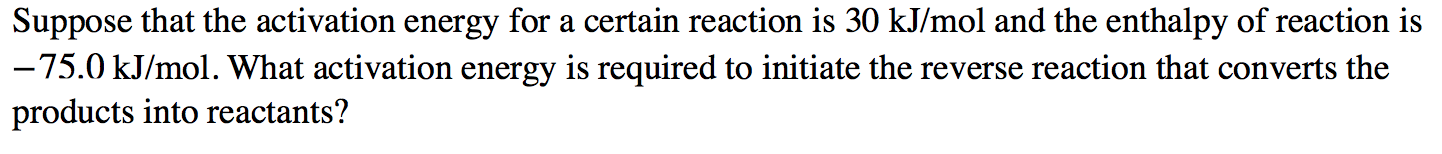Suppose that the activation energy for a certain reaction is 30 kJ/mol and the enthalpy of reaction is –75.0 kJ/mol. What activation energy is required to initiate the reverse reaction that converts the products into reactants?

• ### A reaction has the activation energies of 34.5 and 52.7 kJ/mol for the forward and reverse...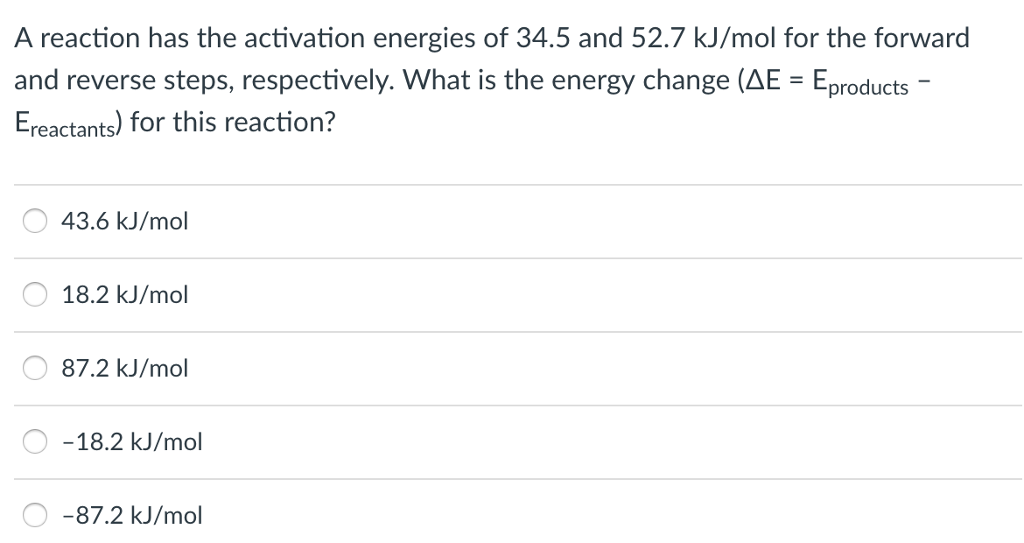A reaction has the activation energies of 34.5 and 52.7 kJ/mol for the forward and reverse steps, respectively. What is the energy change (AE-Eproducts- Ereactants) for this reaction? 43.6 kJ/mol O 18.2 kJ/mol O 87.2 kJ/mol O -18.2 kJ/mol O-87.2 kJ/mol

• ### 1. The forward reaction HOCI → HCIO has an activation energy of 311 kJ/mol. The reverse...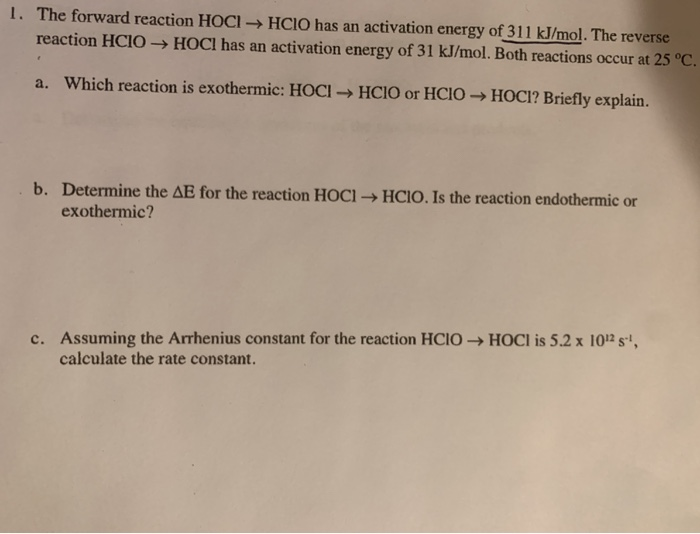1. The forward reaction HOCI → HCIO has an activation energy of 311 kJ/mol. The reverse reaction HCIO → HOCI has an activation energy of 31 kJ/mol. Both reactions occur at 25 °C. a. Which reaction is exothermic: HOCI → HCIO or HCIO → HOCI? Briefly explain. b. Determine the AE for the reaction HOCI → HCIO. Is the reaction endothermic or exothermic? c. Assuming the Arrhenius constant for the reaction HCIO → HOCI is 5.2 x 102 s', calculate...

• ### Question 24 of 30 > A certain reaction has an enthalpy of AH = 34 kJ...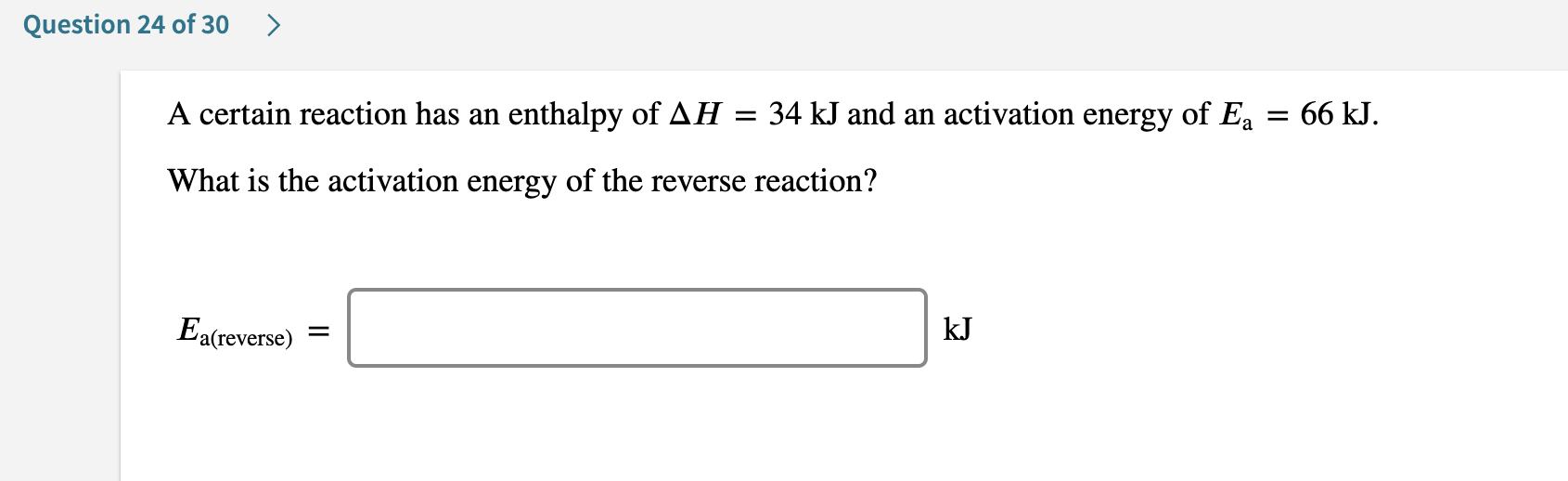Question 24 of 30 > A certain reaction has an enthalpy of AH = 34 kJ and an activation energy of Ea = 66 kJ. What is the activation energy of the reverse reaction? Ea(reverse) = kJ Question 23 of 30 Label the energy diagram for a two-step reaction. A R. + HX Energy RX + H RH + X Reaction coordinate Answer Bank non-limiting transition state rate-limiting transition state products intermediates activation energy starting materials enthalpy change

• ### Certain reaction has an activation energy of 26.64 kJ/mol. At what Kelvin temperature will the reaction...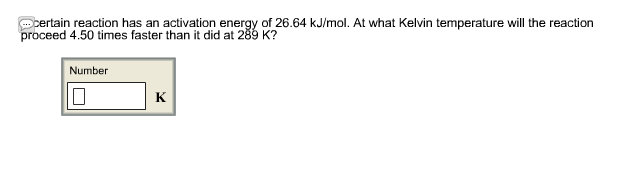Certain reaction has an activation energy of 26.64 kJ/mol. At what Kelvin temperature will the reaction proceed 4.50 times faster than it did at 289 K?

• ### A certain reaction has an activation energy of 48.97 kJ/mol. At what Kelvin temperature will the...

A certain reaction has an activation energy of 48.97 kJ/mol. At what Kelvin temperature will the reaction proceed 6.50 times faster than it did at 295 K?

• ### A certain reaction has an activation energy of 43.60 kJ/mol. At what Kelvin temperature will the...

A certain reaction has an activation energy of 43.60 kJ/mol. At what Kelvin temperature will the reaction proceed 3.00 times faster than it did at 337 K?